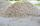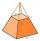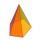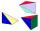# Hexagonal pyramid

Base of the pyramid is a regular hexagon, which can be circumscribed in a circle with a radius of 1 meter. Calculate the volume of a pyramid 2.5 meters high.

Result

V =  2.17 m3

#### Solution:Leave us a comment of example and its solution (i.e. if it is still somewhat unclear...):Be the first to comment!#### To solve this example are needed these knowledge from mathematics:

Tip: Our volume units converter will help you with converion of volume units. See also our trigonometric triangle calculator. Pythagorean theorem is the base for the right triangle calculator.

## Next similar examples:

1. Hexagonal pyramidCalculate the volume and the surface of a regular hexagonal pyramid with a base edge length 3 cm and a height 5 cm.
2. Hexagonal pyramidRegular hexagonal pyramid has dimensions: length edge of the base a = 1.8 dm and the height of the pyramid = 2.4 dm. Calculate the surface area and volume of a pyramid.
3. Gravel - coneMound of gravel has shape of regular circular cone with a height 3.3 meter and a base circumference of 18.85 meters. How many cubic meters of gravel are in the pile? Calculate the weight of gravel if its density is p = 640 kg / cubic m.
4. Tetrahedral pyramidCalculate the volume and surface area of a regular tetrahedral pyramid, its height is \$b cm and the length of the edges of the base is 6 cm.
5. 4side pyramidCalculate the volume and surface of 4 side regular pyramid whose base edge is 4 cm long. The angle from the plane of the side wall and base plane is 60 degrees.
6. Tetrahedral pyramidCalculate the volume and surface of the regular tetrahedral pyramid if content area of the base is 20 cm2 and deviation angle of the side edges from the plane of the base is 60 degrees.
7. Triangular pyramidCalculate the volume and surface area of a regular triangular pyramid whose height is equal to the length of the base edges 10 cm.
8. Triangular pyramidDetermine the volume and surface area of a regular triangular pyramid having a base edge a=20 cm and a lateral edge b = 35 cm
9. Square pyramidCalculate the volume of the pyramid with the side 5cm long and with a square base, side-base has angle of 60 degrees.
10. FlowerbedFlowerbed has the shape of a truncated pyramid, the bottom edge of the base a = 10 m, the upper base b = 9 m. Deviation angle between edge and the base is alpha = 45°. What volume is needed to make this flowerbed? How many plants can be planted if 1 m2 =.
11. PillarCalculate volume of pillar shape of a regular tetrahedral truncated pyramid, if his square have sides a = 19, b = 27 and height is h = 48.
12. Pentagonal pyramidCalculate the volume of a regular 5-side (pentaprism) pyramid ABCDEV; if |AB| = 7.7 cm and a plane ABV, ABC has angle 37 degrees.
13. Mystery of stereometrieTwo regular tetrahedrons have surfaces 88 cm2 and 198 cm2. In what ratio is their volumes? Write as a fraction and as a decimal rounded to 4 decimal places.
14. Truncated pyramidHow many cubic meters is volume of a regular four-side truncated pyramid with edges one meter and 60 cm and high 250 mm?
15. Pyramid a+hCalculate the volume and surface area of the pyramid on the edge and height a = 26 cm. h = 3 dm.
16. PyramidCuboid ABCDEFGH has dimensions AB 3 cm, BC 4 cm, CG 5 cm. Calculate the volume and surface area of a triangular pyramid ADEC.
17. Pyramid in cubeIn a cube with edge 12 dm long we have inscribed pyramid with the apex at the center of the upper wall of the cube. Calculate the volume and surface area of the pyramid.